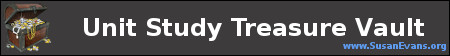LEGO Algebra: Learning Algebraic Formulas

I’ve seen arithmetic done with LEGOs for lower-level elementary math, taking individual LEGO pieces and adding them up, making graphs, and learning about fractions. But LEGO algebra is something I’ve never seen–not until my son Stephen decided that it was possible to write out algebraic equations with white LEGOs on a green base. It’s particularly helpful to write out these algebraic equations to memorize formulas because the tactile component of feeling the LEGOs with your fingers can cause your kinesthetic learners to internalize the formulas more quickly.

The act of building the equations you want to memorize will help you internalize them. My son Stephen has written an explanation for the algebraic equations that he built out of LEGOs:

• In the picture on the left, you have a couple of algebraic systems–each of which is two equations which are related to each other in some way. The system on the top can be solved with the subtraction method by first multiplying both sides of the top equation by 3 and both sides of the bottom one by 2, then subtracting the two equations to remove x and solve for y. (The answer happens to be 2.) We can put the answer for y in one of the equations; let’s do 3x+5y=16, making 3x+5(2)=16. After solving, the answer to system 1 is x=2, y=2.
• The system on the bottom can be solved by substitution with the knowledge that y is equal to the expression x-9. With that information, we can substitute the y in the second equation with x-9, and so solve for x. (The answer is 9.) Then we do the same as we did with system 1 and solve for y (y=x-9    y=9-9    y=0). So the answer is x=9 y=0.
• The picture on the right is three equations which represent various graph shapes. The top one is the equation for a parabola, the middle one is the equation for a circle, and the bottom one is the equation for an ellipse. The yellow dots represent that number or variable raised to the second power (or squared).

Hopefully these explanations can help your high school student understand algebra in a tactile way so that your student is less likely to forget the algebraic formulas. Who knew that LEGO algebra would be possible? My son found a way!Free Bible Crafts e-book!
I agree to have my personal information transfered to MailChimp ( more information )
Join our monthly newsletter for new articles, spiritual encouragment, exclusive printables & free e-courses.
1.Heart of a Philanthropist Blogger Kim says:
•Susan says: